Skip to main content

# 5.6: More Practice with 2D

The following problems involve real samples. Note that you may need to check for peaks due to solvent. Helpful tables may be found here.

Exercise $$\PageIndex{1}$$

The following problems involve real samples. Note that you may need to check for peaks due to solvent. Helpful tables may be found here.Note that the m beside one of the integral values stands for milli (one thousandth). 61.29m = 0.06129 units.

An expansion of the 1H NMR spectrum:Another expansion:A 13C NMR spectrum: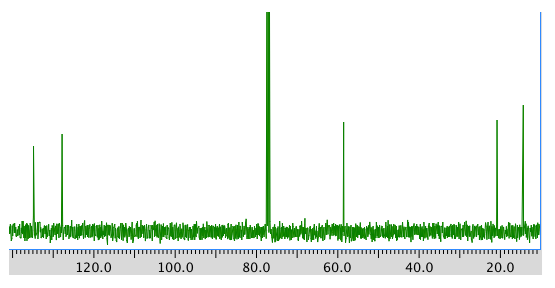COSY spectrum:HMQC spectrum:HMBC spectrum: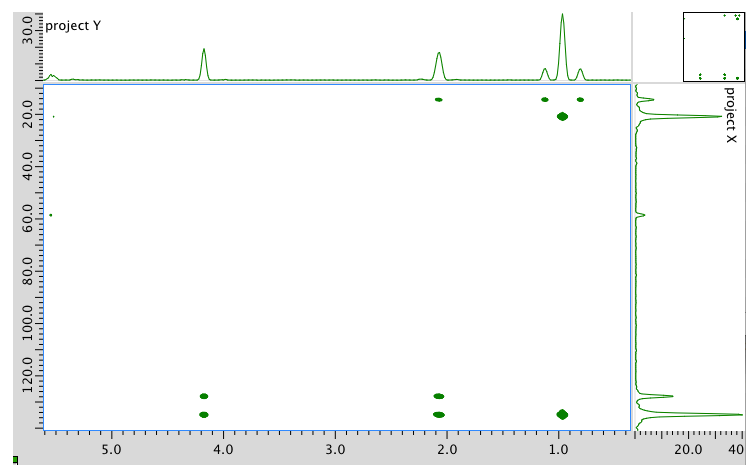Answer

1H NMR

 Chemical shift (ppm) Integration Multiplicity Partial Structure 5.54 2H multiplet CH=C (x 2) 4.17 2H doublet O-CH2-CH 2.08 2H quintet CH-CH2-CH3 1.47 1H boad singlet OH 0.95 3H triplet CH2-CH3

* total # H: 10

13C NMR

 Chemical shift (ppm) Type of carbon 134 sp2 128 sp2 58 sp3-O 21 sp3 14 sp3

*total # C: 5

COSY

 Assignment 1H COSY A 0.95 2.08 B 2.08 0.95, 5.54 C 4.17 5.54 D 5.54 2.08 E 5.54 4.17

*HMQC indicates two hydrogens at 5.54 are in two different environments

HMQC

 Assignment 13C 1H A 14 0.95 B 21 2.08 C 58 4.17 D 128 5.54 E 134 5.54

Formula:

C5H10O (1 O indicated from shift in 13C, 1H NMR)

FW = $$5 \times 12) + (10 \times 1) + (1 \times 16) = 86$$

Compare C5H10 ratio to C5H12 in hydrocarbon

Degrees of unsaturation = $$\frac{(2 \times 5) + 2 - 10}{2} = 1 unit (1 double bond) The data tables should be consitent with this structure: pent-2-en-1-ol (could be cis or trans based on this analysis) Exercise \(\PageIndex{2}$$

Present an analysis of the following data and propose a structure.

MW: 202 amu

The full 1H NMR spectrum in D2O: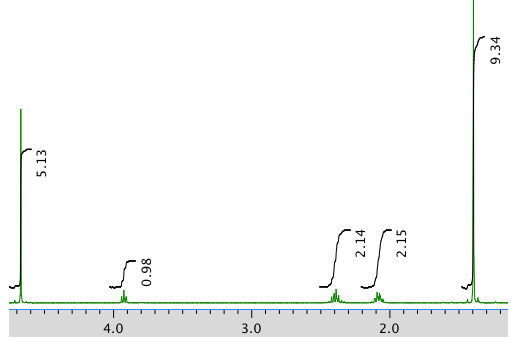An expansion:13C NMR:COSY:Answer

1H NMR:

 Chemical shift (ppm) Integration Multiplicity Partial structure 4.7 5H singlet solvent 3.93 1H triplet CH2-CH-N 2.40 2H multiplet CH2-CH2? 2.09 2H multiplet CH2-CH2? 1.4 9H singlet C(CH3)3

*Total number of H: 19 H

13C NMR:

 Chemical shift (ppm) Type of carbon 170 sp2 (C=O) 80 sp3 (C-O) 52 sp3 (C-N) 32 sp3 28 sp3 26 sp3

*Total number of C: 6 apparent, but two more suggested by symmetry (3 methyl groups in 1H NMR) for 8 C; a third extra suggested by MW fit for 9 C

COSY:

 Assignment 1H COSY Solvent 4.7 -- B 3.93 2.40 D 2.40 2.09 C 2.09 2.40, 2.09 A 1.4 --

Formula:

C9H18O3N2 (extra O indicated from shift in 13C, 1H NMR; second O suggested by C=O in 13C NMR; additional CO needed to fit MW)

FW = $$(9 \times 12) + (18 \times 1) + (3 \times 16) + (2 \times 14) = 202$$

FW = ((9 \times 12) + (18 \times 1) + (3 \times 16) + (2 \times 14) = 202\)

Compare C9H18 to C9H22 for the corresponding hydrocarbon corrected for two nitrogens (therefore two extra hydrogens)

Degrees of unsaturation = $$\frac{(2 \times 9) + 2 + 2 - 10}{2} = 2$$ units (2 double bonds)

The data tables should be consistent with this structure:Exercise $$\PageIndex{3}$$

Present an analysis of the following data and propose a structure.

MW: 164 amu

The full 1H NMR spectrum in CDCl3:An expansion of the 1H NMR spectrum:Another expansion:13C NMR spectrum:COSY spectrum:HMQC:HMBC:Answer

The data should be consistent with this structure: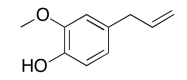Exercise $$\PageIndex{4}$$

Present an analysis of the following data and propose a structure.

MW: 131 amu

The full 1H NMR spectrum in D2O:An expansion:13C NMR:COSY:HMBC:Answer

1H NMR:

 Chemical shift (ppm) Integration Multiplicity Partial Structure 4.71 -- singlet solvent 4.17 1H doublet? CO-CH-N 3.75 3H singlet O-CH3 2.25 1H multiplet CH-CH-(CH3)2 0.92 6H triplet? 2 x CH3

13C NMR:

 Chemical shift (ppm) Type of carbon 170 sp2 C=O 60 sp3 C-N 52 sp3 C-O 30 sp3 C 19 sp3 C

COSY:

 Assignment 1H COSY A 4.17 2.25 B 3.75 -- C 2.25 4.17 D 0.92 2.25

Formula:

C6H13O2N (1 O indicated from shift in 13C, 1H NMR)

FW = $$(6 \times 120 + (13 \times 1) + (2 \times 16) + (1 \times 14) = 131$$

Degrees of unsaturation = $$\frac{(2 \times 6) + 2 + 1 - 13}{2} = 1$$ unit (1 double bond)

The data should be consistent with this structure:Exercise $$\PageIndex{5}$$

Present an analysis of the following data and propose a structure.

MW: 144 amu

The 1H NMR spectrum in CDCl3:An expansion of the 1H NMR spectrum:13C NMR spectrum:COSY:An expansion of the COSY spectrum:HMBC:An expansion of the HMBC spectrum: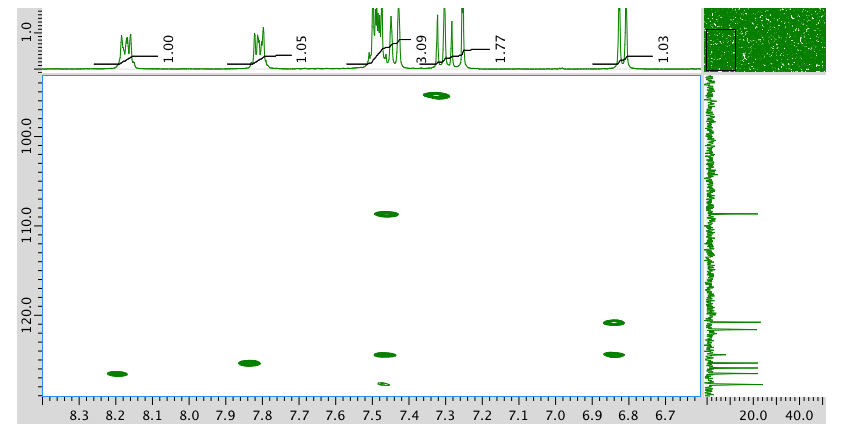AnswerExercise $$\PageIndex{6}$$

Present an analysis of the following data and propose a structure.

MW: 164 amu

The full 1H NMR spectrum in CDCl3:AN expansion:13C NMR:COSY:Expansion of COSY spectrum:Answer

The data should be consistent with this structure:Exercise $$\PageIndex{7}$$

Present an analysis of the following data and propose a structure.

MW: 129 amu

The full 1H NMR spectrum in D2O:13C NMR spectrum: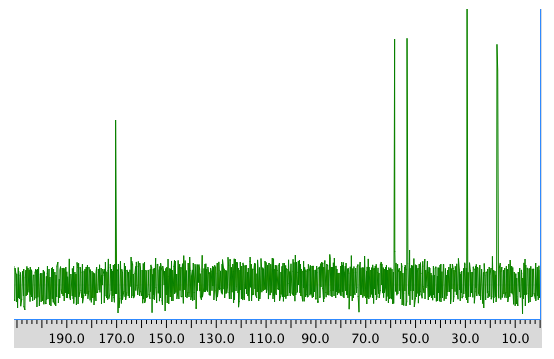COSY spectrum:Answer

The data should be consistent with this structure:Exercise $$\PageIndex{8}$$

Present an analysis of the following data and propose a structure.

MW: 173 amu

The full 1H NMR spectrum in CDCl3:An expansion of the 1H NMR spectrum:13C NMR spectrum:COSY:HMQC:HMBC:Answer

The data should be consistent with this structure:Exercise $$\PageIndex{9}$$

Present an analysis of the following data and propose a structure.

MW: 145 amu

The full 1H NMR spectrum in D2O:An expanded view of 1H NMR spectrum: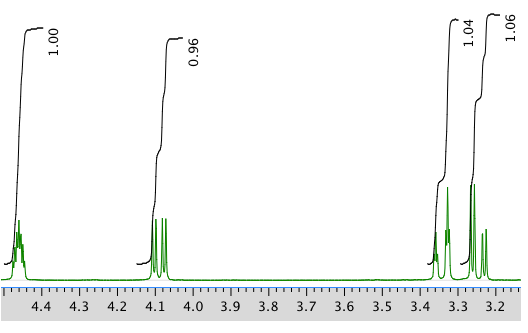Another expanded view:13C NMR spectrum:COSY spectrum:Answer

The data should be consistent with this structure:Exercise $$\PageIndex{10}$$

Present an analysis of the following data and propose a structure.

MW: 251 amu

The full 1H NMR spectrum in DMSO-d6:An expanded view of the 1H NMR spectrum:Another expansion:13C NMR spectrum:COSY spectrum:Expansion of COSY:HMQC spectrum:Answer

The data should be consistent with this structure:Exercise $$\PageIndex{11}$$

Present an analysis of the following data and propose a structure.

MW: 244 amu

The full 1H NMR spectrum in DMSO-d6:An expanded view of the 1H NMR spectrum:Another expansion of the 1H NMR spectrum:13C NMR spectrum:COSY:An expanded view of the COSY spectrum:Answer

The data should be consistent with this structure:Exercise $$\PageIndex{12}$$

Present an analysis of the following data and propose a structure.

MW: 242 amu

The full 1H NMR spectrum in DMSO-d6:Expansion of the 1H NMR spectrum:Another expansion:13C NMR spectrum:COSY:An expansion of the COSY spectrum:Answer

The data should be consistent with this structure:Exercise $$\PageIndex{13}$$

Present an analysis of the following data and propose a structure.

MW: 152 amu

The full 1H NMR spectrum in CDCl3:An expansion of the 1H NMR spectrum:13C NMR spectrum in CDCl3:COSY spectrum: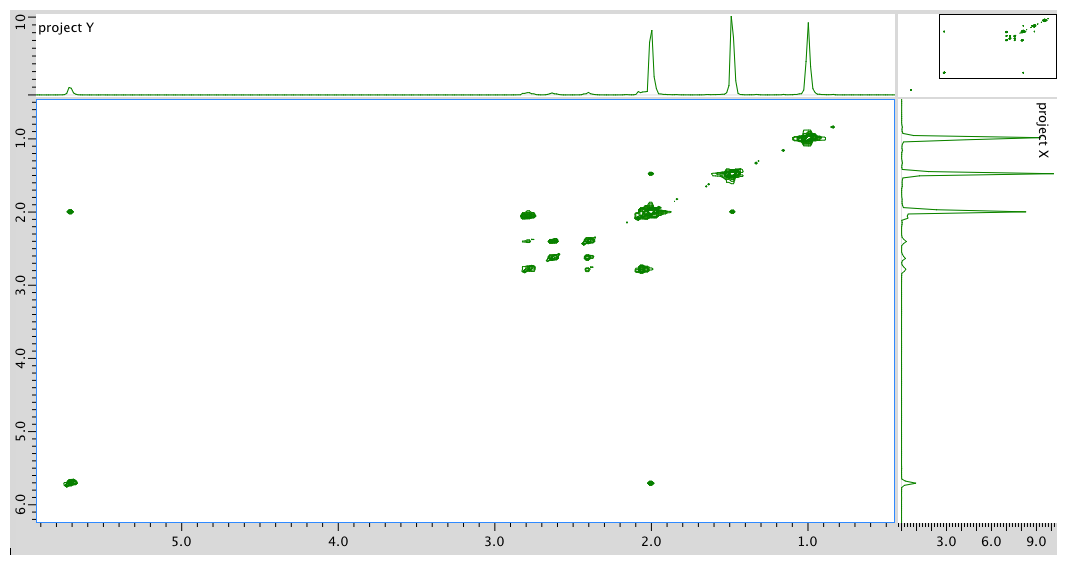HMQC spectrum:HMBC spectrum:Answer

1H NMR:

 Chemical shift (ppm) Integration Multiplicity Partial structure 5.7 1H singlet C=CH-CO 2.77 1H multiplet CH2-CH-CO 2.62 1H multiplet C-CH-C 2.39 1H multiplet C-CH-C 2.05 1H doublet? C-CH-CH? 1.96 3H singlet C-CH3 1.48 3H singlet C-CH3 0.98 3H singlet C-CH3

13C NMR:

 Chemical shft (ppm) Type of carbon 204 sp2 C=O 170 sp2 121 sp2 59 sp3 55 sp3 50 sp3 41 sp3 28 sp3 24 sp3 22 sp3

COSY:

 Assignment 1H COSY 1 2.39 2.77, 2.62? 3 5.7 2.05? 5 2.62 2.62? 7a 2.77 2.05 7b 2.05 2.77 8 1.48 -- 9 0.98 -- 10 1.96 --

Formula:

C10H14O (1 O indicated from shift in 13C, 1H NMR)

FW = $$(10 \times 12) + (14 \times 1) + (1 \times 16) = 150$$

Degrees of unsaturation = $$\frac{(2 \times 10) + 2 - 14}{2} = 4 units (e.g. 2 rings, 2 double bonds) The data should be consistent with this structure:Exercise \(\PageIndex{14}$$

Present an analysis of the following data and propose a structure.

MW: 152 amu

The full 1H NMR spectrum in CDCl3:13C NMR spectrum:COSY spectrum:HMQC spectrum:HMBC spectrum:Answer

The data should be consistent with this structure:Exercise $$\PageIndex{15}$$

Present an analysis of the following data and propose a structure.

MW: 150 amu

The full 1H NMR spectrum in CDCl3: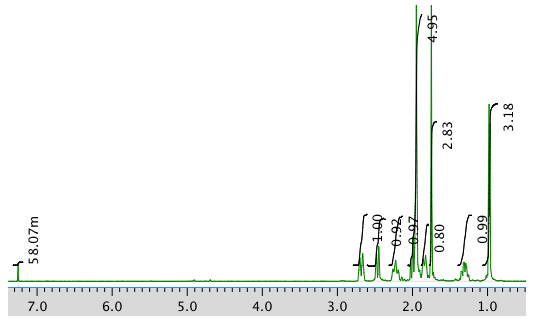An expansion of the 1H NMR spectrum:13C NMR spectrum:COSY spectrum:HMQC spectrum:HMBC spectrum:Answer

The data should be consistent with this structure:NMR spectra obtained on a JEOL 400 MHz NMR spectrometer.